StatLect

# Covariance formula

A covariance formula is an equation used to define or calculate the covariance between two variables.

There are several formulae that can be used, depending on the situation.## General formula

We begin with a general formula, used to define the covariance between two random variablesand:where:

•denotes the covariance;

•denotes the expected value operator.

This is a definition and it is useful because of its generality. However, you need to use the equations below if you need to compute covariance in practice.

## Formula for discrete variables

When the two random variables are discrete, the above formula can be written as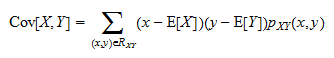where:

•is the set of all couples of values ofandthat can possibly be observed;

•is the joint probability mass function, which gives the probability of observing a specific couple;

• the summation symbolindicates that we need to perform a sum over all the values thatandcan take jointly.

In other words, we sum the products of the deviations of the two random variables from their respective means. Each product is weighted by a probability.

### Example

Suppose that the probability mass function is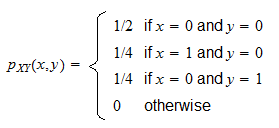The supportcontains three possible couples:The calculations are performed as follows:## Formula for continuous variables

When the two random variables are continuous, the covariance formula involves a double integral: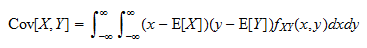where:

•is the joint probability density function ofand;

• both the integrals are betweenand.

### How to compute the double integral

The double integral is computed in two steps:

1. we calculate the inner integral: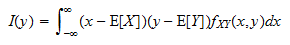which will be found to be a function ofonly becauseis "integrated out";

2. we compute the outer integral### Example

Let the joint probability density function be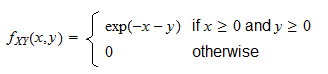In order to compute the expected values, we first need to find the marginal density functions: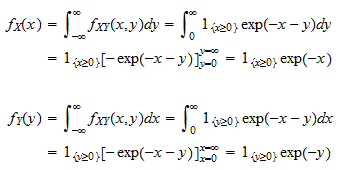We can now work out the covariance: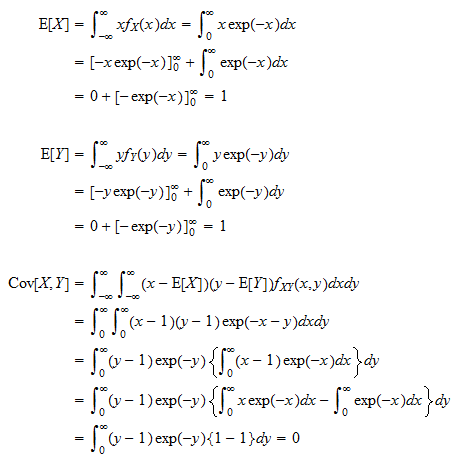## Covariance formula based on moments

Instead of using the formulae above to find the covariance, it is often easier to use the following equivalent equation based on moments and cross moments:### Example

In the previous example, after finding the expected values ofand, we could have done: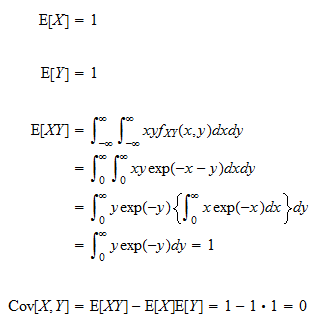### Use with moment generating function

When we know the joint moment generating function ofand, we can use it to compute the moments,andand then plug their values in the formula above.

## Formulae for the sample covariance

Until now, we have discussed how to calculate the covariance between two random variables.

However, there is another concept, that of sample covariance, which is used to measure the degree of association between two observed variables in a sample of data.

Givenobserved couplestheir sample covariance is calculated aswhereandare the sample means of the two variables:### Unbiased sample covariance

An alternative to the formula above is the so-called unbiased sample covarianceThe only difference is that we divide byinstead of dividing by.

If theobserved couples are independent draws from the joint distribution of two random variablesand, thenis an unbiased estimator of.

### Example

In this example, there are four observed couples, whose values are reported in the columns of the table below.

The last two rows of the table are used to calculate the means and the sample covariance (biased and unbiased).

Observation number xj Deviation of xj from mean yj Deviation of yj from mean Product of deviations
1 1 -1 5 2 -2
2 3 1 0 -3 -3
3 0 -2 -1 -4 8
4 4 2 8 5 10
Sum 8 0 12 0 13
Divide sum by n 2 3 13/4
Divide sum by n-1 13/3

## More details, proofs and exercises

More details about these formulae - including proofs and solved exercises - can be found in the lecture on Covariance.

## Keep reading the glossary

Previous entry: Countable additivity

Next entry: Covariance stationary